Monday , May 23 2022# Electricity: 10th CBSE Science Physics

#### Question: (1). How is the direction of electric current related to the direction of flow of electrons in a wire? (2). Calculate the current in a circuit, if 500 C of charge passes through it in 10 minutes.

1. The direction of electric current is opposite to the direction of flow of electrons in a wire.
2. Given   Q = 500 C,
t = 10 minute
= 10 × 60 = 600 s, I = ?
Using the relation I = Q/t = 500/600 = 0.83 A

#### Question: Define electric current and state its SI unit. With the help of Ohm’s law explain the meaning of 1 ohm resistance.

Answer: It is defined as the rate of flow of electric charge through an electric circuit.
Its SI unit is ampere (A).
Ohm’s law is V = IR
Let V = 1 ohm and I = 1 ampere, then R = 1 volt / 1 ampere = 1 ohm

Thus, 1 ohm is the resistance of a conductor, if under the effect of a potential difference of 1 volt across it a current of 1 ampere flows through it.

#### Question: (a). What is the total resistance of n resistors each of resistance ‘R’ connected in: (i) series? (ii) parallel? (b). Calculate the resultant resistance of 3 resistors 3 Ω, 4 Ω and 12 Ω connected in parallel.

(a) In series combination, we have
Rs = R1 + R2 + …. to n = nR
In parallel combination, we have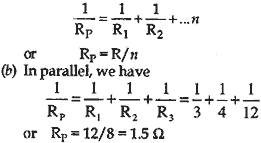#### Question: (a) State Ohm’s law. Express it mathematically. (b) Write symbols used in electric circuits to represent: (i) variable resistance (ii) voltmeter. (c) An electric bulb is rated 220 V and 100 W. When it is operated on 110 V, what will be the power consumed?

Answer: (a) See Chapter at a Glance.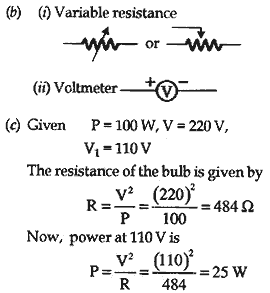#### Question: Two conducting wires of same material, equal length and equal diameter are connected in series. How does the heat produced by the combination of resistance change?

Answer: Let the resistance of the wire be R.
When connected in series, the total resistance is
Rs = R + R = 2R
Heat produced by series combination
H = V²/2R × t
Heat produced by individual resistors
H’ = V²/R × t
Therefore,
H’ = H/2

#### (i) State the type of combination of the two resistors in the circuit. (ii) How much current would flow through: (a) 10 Ω resistor and (b) 15 Ω resistors? (iii) What would be the ammeter reading?

(ii) (a) I1 = V/R1 = 3/10 = 0.3 A
(b) I2 = V/R2 = 3/15 = 0.2 A
Current flowing in the circuit = 0.3 A + 0.2 A = 0.5 A.
(iii) Ammeter reading is 0.5 A.

#### Question: (1) Why are copper and aluminium wires generally used for electrical transmission and distribution purposes? (2) Two wires, one of copper and other of manganin, have equal lengths and equal resistances. Which wire is thicker? Given that resistances. Which wire is thicker? Given that resistivity of copper is lower than that of manganin.

1. They have low resistance, so very small amount of energy is wasted in transmission. They are ductile so can be easily drawn into wires.
2. From the expression R = ρL/A , we find that A ∝ ρ.

Resistivity of manganin is very large, so it is thicker than copper.

#### Question: (a) Nichrome wire of length L a radius ‘R’ has resistance of 10 Ω. How would the resistance of the wire change when: (i) only length of the wire is doubled? (ii) only diameter of the wire is doubled? Justify your answer. (b) Why element of electrical heating devices are made-up of alloys?

Answer: (a) (i)     R ∝ L
So resistance becomes two times,
i.e.,      R = 2 × 10 = 20 Ω.
(ii) R ∝ 1/A ∝ 1/D²
So when diameter is doubled, resistance
becomes 1/4 of its original,
i.e.,      R = 10/4 = 2.5 Ω

(b) This is because alloys do not oxidize readily at high temperature.

#### Question: Resistivity of two element A and B are 1.62 × 10-8 Ω m and 520 × 10-8 Ω m respectively. Out of these two, name the element that can be used to make: (i) filament of electric bulb. (ii) wires for electrical transmission lines. Justify your answer in each case.

Answer: (i) Element B: It has more resistivity (520 × 10-8 Ω m).
(ii) Element A: It has less resistivity and hence less heating effect/ dissipation of energy during transmission of power.

#### Question: State Ohm’s law. Calculate the resistance of a conductor, if the current flowing through it is 0.2 A when the applied potential difference is 0.8 V.

Answer: It states that physical conditions remaining same the electric current flowing through a metallic conductor is directly proportional to the potential difference across its ends.
Given I = 0.2 A, V = 0.8 V, R = ?
We have R = V/I 0.8/0.2 = 4 ohm

#### Question: State reasons for the following: (1). Tungsten is used for making bulb filaments. (2). Copper and aluminium are generally used for electricity transmission. (3). Alloys are commonly used in electrical heating devices such as electric iron and toaster.

1. Tungsten is a metal which has a very high melting point and comparatively high resistivity, therefore, it used in making filament of bulbs.
2. Both copper and aluminium are good conductors of electricity because they have comparatively low resistivity, i.e., these metals offer low resistance to the flow of electric current.
3. It is because resistivity of alloys is generally higher than that of its constituent metals.

#### Question: Draw the nature of V-I graph for a nichrome wire. (V – Potential difference, I – Current) A metallic wire of 625 mm length offers a  4 Ω resistance. If resistivity of the metal is 4.8 × 10-7 ohm-meter, then calculate the area of cross-section of the wire.

Answer: The graph is as shown.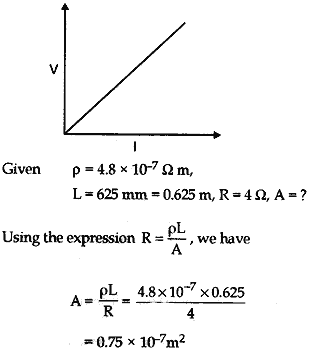#### (i) Which of the two circuits that has more resistance? (ii) Through which circuit more current passes? (iii) In which circuit, the potential difference across each resistor is equal? (iv) If R1 > R2 > R3, in which circuit more heat will be produced in R1 as compared to other two resistors?

♦ It is defined as the amount of work done in moving a unit charge i.e., V = W/q.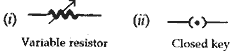(b) (i) Circuit (I) has more resistance.
(ii) More current passes through circuit (II).
(iii) Same potential difference across each resistor in circuit (II).
(iv) More heat will produce in R1 in circuit (I).

#### Question: When a high resistance voltmeter is connected directly across an electric bulb, its reading is 2 V. An electric cell is sending the current of 0.4 ampere (measured by an ammeter) in the electric circuit. (a) Draw the circuit. (b) Find the resistance of the electric bulb. (c) State the law that is applied for making these calculation. If a graph is plotted between V and I, show the nature of the graph obtained.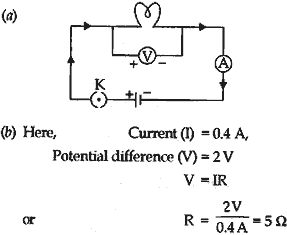(c) Ohm’s law is applied. The V-I graph will be a straight line that passes through the origin of the graph.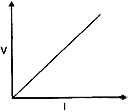#### Answer the following question in relation to them: (i) Among silver and copper which one is better conductor? Why? (ii) Which material would you advise to use in electrical heating device? Why?

1. Potential different between two points is said to be one volt when one joule of work is done to move a charge of one coulomb from one point to the other.
2. Heating element glows because its resistivity of the connecting cord.
3. (i) Silver is a better conductor because it has the less resistivity.
(ii) Nichrome, because its resistivity is much higher in comparison to the others.

#### Question: Draw a schematic diagram of an electric circuit (in the “on” position) consisting of a battery of five cells of 2 V each, a 5 Ω resistor, a 12 Ω resistor and a plug key, all connected in series. An ammeter is put in the circuit to measure the electric current through the resistors and a voltmeter is connected so as to measure the potential difference across the 12 Ω resistor. Calculate the reading shown by the: (a) ammeter (b) voltmeter in the below electric circuit.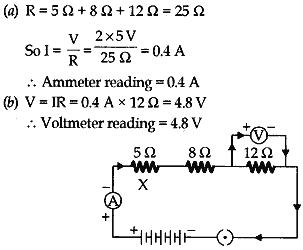#### Question: (a) Explain how does a cell maintain current in a circuit. (b) In the circuit given below the resistance of the path xTy = 2 Ω and that of xZy = 6 Ω. (i) Find the equivalent resistance between x and y. (ii) Find the current in the main circuit. (iii) Calculate the current that flows through the path xTy and xZy.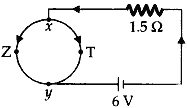Answer: (a) The chemical action within a cell generates the potential difference across the terminals of the cell. This potential difference sets and maintains current in the circuit.
(b) (i) Equivalent resistance, 1/Re = 1/2 + 1/6
= 3+1/6 = 4/6 = 2/3
or Re = 1.5 Ω

(ii) Total resistance of the circuit
= Re + 1.5 Ω
= 1.5 Ω + 1.5 Ω = 3 Ω
∴  Current (I) = 6 V/3 Ω = 2 A

(iii) P.D. across the parallel combination of 2 Ω and 6 Ω = 1.5 × 2 = 3 V
∴ Current through 2 Ω resistance = 3 V/6 Ω = 0.5 A

## Sources of Energy: 10th Science Chapter 14

Class: 10th Class Subject: Science Chapter: Chapter 14: Sources of Energy Quiz: – Questions MCQs: …